# CBSE Class 9 Science Work Energy And Power Notes Set B

WORK, ENERGY AND POWER

INTRODUCTION

In everyday language, the word work is used to describe any activity in which muscular or mental effort is exerted. In physical, the word work has a special meaning. Work in one done when the force acting on body produced motion in it in the direction of force (or in the direction of component of force). Thus a boy pushing the wall is doing no work from physics’ point of view. If is because the force exerted by the body is not producing motion of the wall. The speed at which work can be done is an indication of the power of the body doing work. For example, a boy may carry a suitcase upstairs in 3 minutes while a man may do it in 1 minute. Obviously, the power of the man is more than the power of the boy. Thus, time factor is important for power. A body which has the capacity to do work is said to posses energy. The greater the capacity of a body to do work, the greater the energy it has. Thus work, energy and the power are related to each other. In this topic we shall deal with these three important concepts of physics.

WORK

In our day to day life, the word work means any kind of mental and physical activity. For example, we say that we are doing work while,

(ii) cooking the food,

(iii) walking on a level road with a box on our head,

(iv) pushing a wall of a house but fails to do so.

In all these cases, either mental or physical activity is involved.

But is physics, the term work has entirely a different meaning. In physics work is done if a force applied on a body displaced the body in its own direction. In other words, the condition which must be satisfied for the work done are : (i) a force must act on the body and (ii) the body must be displaced from one position to another position. Thus, no work is done in all cases mentioned above.

Definition :

Work is said to be done by a force on a body o an object if the force applied causes a displacement in the body or object.

Eg. : Work is done, when a box is dragged on the floor from one position to another. In this case, force is on box to drag it one the floor and the box moves through a certain distance between one position to another position.

(a) Measurement of Work :

Work is measured by the product of force and the displacement in the direction of force. Work is a scalar quantity.

Work = Force × displacement in the direction of forceFrom equation (i)

W = Fd cosθ = Fd cos00

So, W = Fd (maximum)

When force and displacement are in same direction then work done will be maximum.

Case - II : When  = 900, then -

From equation (i)       cos 00=1

W = Fd cos900

So, W =0When force and displacement are perpendicular to each other then work done will be zero.

Eg. : If a body is moving in horizontal direction then work done by the force of gravity will be zero.Eg. : if a body is moving on a circular path then work done by the centripetal force will be zero, because the direction of centripetal force is towards the center of the circle and displacement will be along the tangent.Case III :

If  = 1800

then from equation (i)

W = Fd  cos 180                               cos 1800 = -1

then   W = - Fd

When the force and displacement are in opposite direction then work done will be negative.

Eg.: When a spring is compressed then the force applied by the spring and the displacement will be in opposite direction to each other, so work done by the spring will be negative. When the spring is stretched then the work done will also be negativeEg.: When a body of mass m in lifted upward a fore F = mg has to be applied upward

Work done by the force of gravity will be negative

Work done, W = - mgh(b) Units of Work done :

Work done, W = Fd

In C.G.S. system the unit of work done is dyne x cm = erg.

Definition of 1 etg :

If F = 1 dyne and d = 1 cm.

then W = 1 × 1 = 1 erg.

If one dyne force is applied on a body and displacement in the body in 1 cm in the direction of force, then work done will be one erg.

S.I. unit of work done is Newton × metre = joule.

Definition of 1 joule :

if F = 1N and       d = 1m.

then, W = 1 × 1 = 1 joule (J)

If a force of 1 Newton is applied on a body and displacement in the body is 1m in the direction of force then work done will be 1 joule.

Relation between joule and erg :

1 joule = 107 erg

NOTE :

(i) If F = 0 then work done, W = 0

Eg. A student revising his notes by memory without moving his limbs is doing no physical work.

A meditating saint is doing no physical work though he keeps sitting for hours.

(ii) If displacement, d = 0 then work done, W = 0.

Eg. A foolish labour trying to displace a building has done no work though he may spend the whole day.

Erg and joule are the absolute units of work done.

Gravitational unit of work :

Work is said to have gravitational unit of work if unit gravitational force displaces the body through unit distance in the direction of force.

(i) In C.G.S. system, gravitational unit of work is gram-weight-centimeter ( g wt cm).

Since W = FS

1g wt cm = 1 g wt × 1 cm = 981 dyne × 1 cm

1g wt cm = 981 erg.

Thus 1g-wt-cm of work is done when a force of 1g-wt displaces a body through 1 cm in its own direction.

(ii) In S.I. system, gravitational unit of work is kilogram weight meter (kg wt m)

1kg wt m = 1kg wt × 1m = 9.81 N  × 1 m

1 kg wt m = 9.81 J

Thus, 1 kg wt m of work is done when a force of 1 kg-wt displaces a body through 1 m in its own direction.

(c) Positive Work done :

When the angle between force and the displacement is acute (), then work done will be positive because one component of force (F cos) is in the direction of displacement so work done by this component will be positive (Fd cos). Work done by the vertical component (i.e. F sin) will be zero (the angle between F sin and displacement is 900) so net work done will be positive.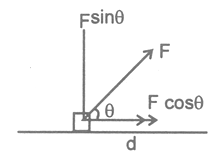(i) In lifting a weight upward by applying an upward force, the work done by the applied force will be positive.

(ii) In stretching a spring, the work done by the eternal force will be positive.

(d) Negative Work done :

When the angle between the force and the displacement is obtuse, (), then work done will be negative because work done by the horizontal component of force (i.e. F cos) is negative (-Fd cos) and the work done by the vertical component (F sin) will be zero, so net work done will be negative.ILLUSTRATIONS

1.A porter lifts a luggage of 15 kg from the ground and put it on his head, 1.5 m above the ground. Calculate the work done by him on the luggage. (take g = 10 m/s2.)

Sol. Mass of luggage, m = 15 kg

displacement, d = 1.5 m

acceleration due to gravity, g = 10 m/s2

work done, W = Fd = mgd

W = 15 × 10 × 1.5 = 225 J

2.A force of 10 N displaces a body by 5m, the angle between force and displacement i 600, then find the work done.

Sol.   Force, F = 10 N,

displacement, d = 5m,

angle between force and displacement, θ = 600,

work done, W = Fd cos = 10 × 5 × cos600,

then,       W = 10 × 5 ×  1/2         W = 25 J

ENERGY

When a man does a work, he feels tired. he feels that he has lost something which he must regain to work more. A weak man gets exhausted after doing only a small amount of work. A strong man can continue to work for longer duration.

Something that a working man loses is called energy.

Definition : Capacity of doing work or total work done by a man or by an agent, is called the energy of the man or the agent.

(a) Units of energy :

C.G.S. unit of energy is erg and S.I. unit of energy is joule.

NOTE :

(i) kilo Watt × hour (kWh) is commercial unit of energy.

1 kWh = 1000 watt × 60 × 60 s.

= 3.6 × 106 watt × s

1 kWh = 3.6 × 106 J.

(ii) Electron volt (eV) is also the unit of energy. The energy of an electron, when it is accelerated by a potential difference of 1 volt, is known as one eV

1 eV = 1.6 × 10-19 J.

DIFFERENCE FORMS OF ENERGY

(i) Heat energy :

When we burn coal, wood or gas, heat energy is released. Steam possess heat energy that is why is a steam engine, the heat energy of steam is used to get the work done. Sun also radiates hat energy.

(ii) Light energy :

It is a form of energy which gives us the sensation of vision. Natural source of light is the sun. An electric bulb also emits light energy.

(iii) Sound energy :

The energy emitted by a vibrating wire, tuning fork, vibrating membrane etc., that can be sensed by human ears is called sound energy.

Eg. whistle, flute, sitar, all emits sound energy when they are made to vibrate.

(iv) Magnetic energy :

A magnet also possess energy known as magnetic energy. When a current is passed through a coil, it stores magnetic energy.

(v) Electrical energy :

An electric cell stores electrical energy. Two changes placed at some distance experience a force. They also possess electrical energy.

Eg. A charged body possess electrical energy.

(vi) Solar energy :

The energy radiated by the sun is solar energy. Sun is the natural source of energy.

(viii) Nuclear energy :

Sometimes, a heavy nucleus breaks into two or more lighter nuclei with the release of some energy. This energy is called nuclear energy and the process is called nuclear fission. On the other hand, when two lighter nuclei combine to form a heavy nucleus, the process is called nuclear fusion.

KINETIC ENERGY

Energy of a body due to its motion is known as the kinetic energy of the body. If a body of mass m is moving with velocity v, then its kinetic energy = 1/2 mv2.

(a) Dedication of Formula for K.E. :

The kinetic energy of a moving body can be find by calculating the work done in bringing the body in motion from rest.

A body of mass m is moving with initial velocity u. A force F is applied in the direction of motion then after some distance s, its final velocity becomes v.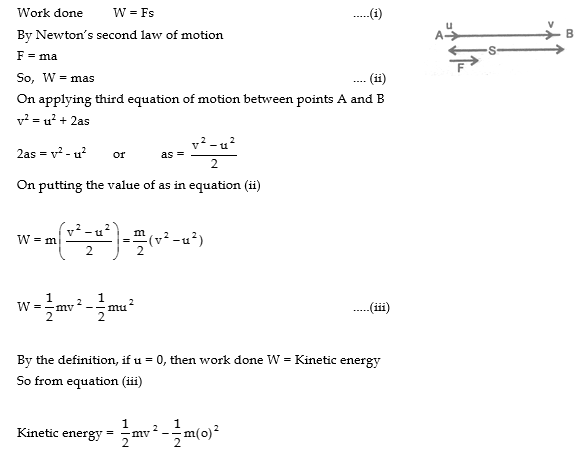3.What is the work to be done to increase the velocity of a car from 30 km/h to 60 km/h. If mass of the car is 1500 kg.

Sol. Mass of car, m = 1500 kg.

Initial velocity, u = 30 km/h = 8.33 m/s.

Final velocity, v = 60 km/h = 16.67 m/s.

Work done,  W= 1/2 mv2 - 1/2 mu2

W = 1/2 × 1500[(16.67)2 - (8.33)2]

= 750(277.9 - 69.4)

W = 750 × 208.5 = 156375 J.

W = 1.56 × 105 J.

POTENTIAL ENERGY

When a child lifts a football from the ground and place it on the top of a table, some work is done on the ball. Now, if this football falls down from the top of the table and hits another football lying on the ground, then the football lying on the ground in displaced from its position. This simple activity shows that a falling football is able to do work.

“We know, anything capable of doing work possess energy.” Therefore a football placed on the table also possess energy. This energy of the football lying on the top of the table is known as potential energy. Now the question arises from where this potential energy came in the football lying on the top of the table. Infect, the work done by the child to rises it to the top of table from the ground the stored as energy. This stored energy is known as potential energy.

(a) Definition of Potential Energy :

The energy possessed by a body virtue of its positions or shape or configuration is known as potential energy.

(b) Examples :

(i) Water stored in dam has potential energy due to its position.

(ii) A stone lying on the top of all hill or a mountain has potential energy due to its position.

(iii) A stretched or compressed spring has potential energy due to this shape. When spring is stretched or compressed, work is done on it. This work done is stored as potential energy of the stretched or compressed spring.

(iv) A wound spring of a watch has potential energy due to its shape.

(v) A stretched bow and arrow has potential energy due to its shape.

(c) Gravitational and Elastic Potential Energy :

(i) Gravitational potential energy :

The energy possessed by a body by virtue of its position (i.e., height above the surface of the earth) is known as gravitational potential energy.

(ii) Elastic potential energy :

The energy possessed by a body by virtue of its deformed shape (i.e. either stretched or compressed) is known as elastic potential energy.

(d) Expression for Potential Energy of A body at a Certain Height :

The energy possessed by a body due to its position in the gravitational field of the earth is called gravitational potential energy.

Consider a block of mass m which is to be raised to a height ‘h’. The force required to lift the block must be equal to the gravitational force (i.e. weight of the block). Thus, Fg = mg. Let the applied force on the block be F = mg and the block is raised to the height h as shown in the figure.Work done by the applied force F is given by

Work done against the gravitational force on the block is known as gravitational potential energy.

Ug= -(mgh) = mgh

IMPORTANT INFORMATION :

(i) Gravitational potential energy of a body on the surface of the earth (i.e. h = 0) is zero.

(ii) Gravitational potential energy of a body increases if the body moves upward (i.e. h increases).

(iii) Gravitational potential energy of a body deceases if the body moves downward (i.e. decreases).

(iv) Gravitational potential energy depends only on the initial and final position of the body and not on the path followed by the body to go form initial position to final position. It means, the gravitational potential energy of body at height h will be same if it is either taken straight upward to height h or it is taken along a curved path to height h.

INTERCOVERSION OF POTENTIAL AND KINEIC ENERGY

(i) For a freely falling body, potential energy changes into kinetic energy.

Let a body of mass m be at rest at a point at height h from the ground.

At highest point :

Potential energy of the body          U1 = mgh

Kinetic energy of the body              K1 = 0                    [u = 0]

As the body falls freely, it gains velocity and reduces height. Let the body have velocity v when it reaches the ground.

At lowest point :

(ii) For an upward projected body, kinetic energy changes into potential energy.

Let a body of mass m be projected upwards with a velocity u from a point on the ground.

At lowest point :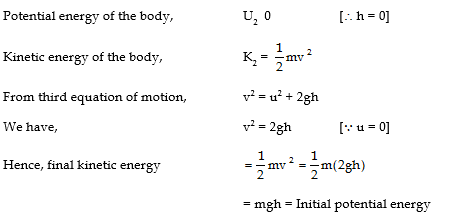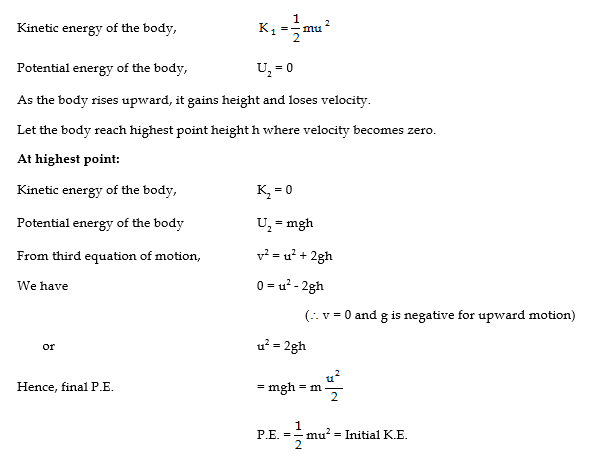LAW Of CONSERVATION OF ENERGY

According to this principle, the total sum of energy of all kinds in an isolated system remains constant at all times. This means that energy can neither be created nor be destroyed. Energy can only be changed from one form to another form of energy. The amount of energy appearing in one form is always equal to the amount of energy disappearing in some other form. The total energy thus remains constant, always provided, at all point, we measure the amount of energy present in each from (including mass which too is a form of energy).

(a) Law of Conservation of Mechanical Energy :

If conservation forces are acting on a body or on a system, then the sum of kinetic energy and potential energy (mechanical energy) of the body or of the system will be conserved. If the presence of conservative forces, if the kinetic energy is increased by an amount of  then the potential energy of the body will decrease by the same amount i.e.Δ U.Final mechanical energy = Initial mechanical energy.

So in the presence of conservative forces the sum of Kinetic energy and the potential energy of a body will be conserved.

(b) Mechanical Energy of a Freely Falling Body:

Let a body of mass m is at rest at a height h from the earth’s surface, when it starts falling, after a distance x (point B) its velocity becomes v and at earth’s surface its velocity is v’/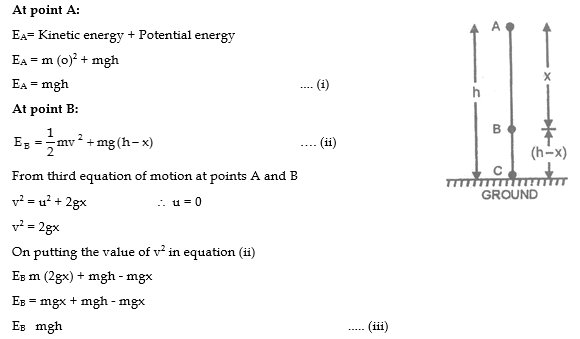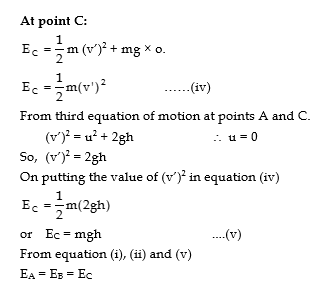Hence, the mechanical energy of a freely falling body will be constant.

i.e. Total energy of the body during free fall, remains constant at all positions. The form of energy, however keeps on changing. AT point A, energy is entirely potential energy and at point C, it is entirely kinetic energy In between A and C, energy is partially potential and practically kinetic. This variation of energy is shown in figure. Total mechanical energy stays constant (mgh) throughout.Thus is an isolated system, where only conservation forces cause energy changes, the kinetic energy and potential energy can change, but the mechanical energy of the system (which is sum of kinetic energy and potential energy) cannot change. We can, therefore, equate the sum of kinetic energy and potential energy at one instant to the sum of kinetic energy and potential energy at another instant without considering intermediate state. This law has been found to be valid in every situation. No violation, whatsoever, of this law has ever been observed.ILLUSTRATION

1.A body of mass 10 kg is kept at a height 10 m from the ground, when it is released after sometime its kinetic energy becomes 450 J. What will be the potential energy of the body at the instant ?

Sol. At a height of 10 m. The mechanical energy of the body,

E = Kinetic energy + potential energy

E = m (o)2 + mgh                               (initial velocity of the body is zero)

E = 10 × 10 × 10 = 1000 J.

After sometime the kinetic energy is 450 J. Suppose at that instant potential energy is U, then by the law of conservation of mechanical energy.

E = 450 + U

1000 = 450 + U

or U = 1000 - 450            U = 550 J.

SOME OTHER EXAMPLES OF CONSERVATION OF ENERGY

(i) Vibrations of a simple pendulum :

In the figure OA is normal position of rest of a simple pendulum. When the bob of the pendulum is displaced to B, through a height h, it is given potential energy (mgh), where m is the mass of the bob. On releasing the bob at B, it moves towards A. Potential energy has been converted into kinetic energy. The bob, therefore, cannot stop at A. On account of inertia, it overshoots the position A and reaches C at the same height h above A. The entire kinetic energy of the bob at A is converted into potential energy at C. The whole process is repeated and the pendulum vibrates about the equilibrium position A. At extreme positions B and C, the bob is momentarily at rest. Therefore its kinetic energy is zero. The entire energy at B and C is potential energy. At A, there is no height and hence no potential energy. The entire energy at A is kinetic energy(ii) Motion of a small spherical ball over a watch glass :

Figure shows a watch glass of a large concave mirror embedded in clay. Consider a tiny spherical ball placed at the edge B at a height h above the centre A. if m is mass of the ball, potential energy of the ball held at B is mgh.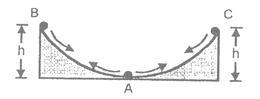When the ball is released from point B, it starts rolling down the mirror. Potential energy of the ball is being converted into kinetic energy. At the bottom A, velocity of the ball is maximum as the entire

potential energy has been converted into kinetic energy. The ball cannot stop at A on account of inertia. It goes over to the other edge C. The velocity of the ball goes on decreasing and so does its kinetic energy. AT point C kinetic energy is zero and potential energy is maximum. The entire process is repeated at thus the ball keeps on rolling over the mirror about A.

NOTE :

In all the above examples, we have neglected the loss of energy due to  air resistance/friction etc. If we were to take into account these opposing forces, kinetic energy would go on decreasing as it appears in the form of heat energy. But total energy (including the heat energy) would remain constant.

POWER

Introduction:

We have learnt that when a force causes displacement, work is done. Work done is measured as the product of the magnitude of the force and the displacement in its direction.

A certain amount of work done appears to be tiring if done quickly and in a very short time. Same amount of work is done slowly in a larger interval of time gives no feeling of tiredness.

This fact has given rise to a new concept i.e. the rate at which work is done ant it defines power.

Definition :

Rate of doing work i.e. work done per unit time (second) by a man or machine, is called power of the man or the machine. it is represented by the symbol P. It is a scalar quantity.

(a) Expression for Power :

Let a force F displaces a body by distance S in its own direction in time t, to give it a velocity, v=S/t

Then, by definition -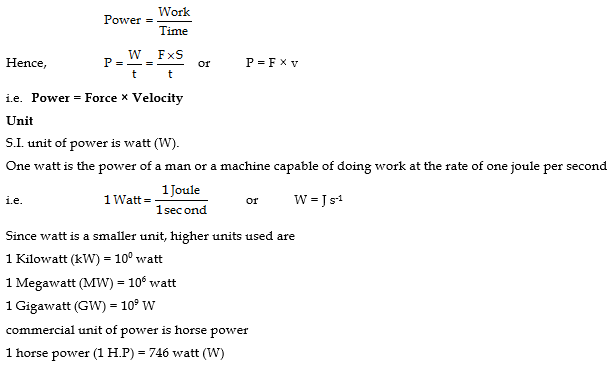(b) Distinction with Energy :

Energy measures total work done.

Power measures work done per unit time (second).

Eg : An old man works slowly for eight hours and manufactures 24 items in a day. His younger son works quickly for two hours and manufactures 16 items in a day.

The old man has more energy but less power.

The young mas has less energy but more power.

ILLUSTRAION

1. A boy of mass 50 kg runs up a staircase of 45 steps in 9 s. If the height of each step is 15 cm. Find his power. (g = 10 m/s2)

Sol. Mass of man, m = 50 kg.

Height covered, h = 45 × 15 = 675 cm = 6.75 m

Power P =W/ t = mgh / t= 50x10x6.75 /9

P = 375 watt.

ENERGY FROM THE SUN

The sun is the ultimate source of all forms of energy available on the earth. This can illustrated as follows:

(a) Wind Energy:

Solar energy heats up the surface of the earth and the air near it. The hot air rises up and the cool air from above rushes to occupy its space. This makes the air to move. Moving air is known as wind and possesses kinetic energy. Thus, solar energy + air  wind energy. Wind energy is converted into electrical energy in a wind farm using wind mills.

(b) Green Plants make their food:

Green leaves of plants make their food using sunlight (i.e. sun energy) by the process of photosynthesis. The cells of green leaves of plants contain chloroplasts. Each chloroplast contains chlorophyll (a green pigment) which converts carbon dioxide into sugar in the presence of sunlight by the process of photosynthesis. Process of photosynthesis is represented as follows:The energy stored in the food is known as chemical energy. The food eaten by a man or an animal provides him the muscular energy, is used to do work.

In Solar energy  + Green leaves→  Food (chemical energy) Muscular energy  Mechanical energy (work)

## Tags:

Click for more Science Study Material

## Latest NCERT & CBSE News

Read the latest news and announcements from NCERT and CBSE below. Important updates relating to your studies which will help you to keep yourself updated with latest happenings in school level education. Keep yourself updated with all latest news and also read articles from teachers which will help you to improve your studies, increase motivation level and promote faster learning

### TV Channels for Students by CBSE

In enhancing the students studying part, the Government is planning to introduce one standard one channel plan. The lockdown in India has adversely collapsed all the operations including schools, colleges, and workplaces with unanticipated setbacks. On behalf of the...

### CBSE Board Exams in July Press Release

CBSE has released press note for the dates for the CBSE Board exams for Class 10. CBSE said “With regards to conduct of Class X examinations several queries are being received by the CBSE. In this context, it is once again reiterated that remaining examinations of...

### Checking of Boards Answer sheets starts today

CBSE has instructed almost 3000 schools to start the evaluation work for class 10th and class 12th board exam answer sheets from today. The checking of answer sheets for class 10th and class 12th was stopped earlier due to covid crisis in the country. Now in order to...

### Art Integrated Project work for classes 1 to 10

CBSE Board has decided to introduce Art-Integrated Project work for classes I to X to promote Art-Integrated Learning in schools to make teaching learning Competency-Based and joyful. As part of this, at least one Art-Integrated Project in each subject shall be taken...

### Class 12 Board Exams Datesheet Announced

CBSE has announced the datesheet of the remaining class 12 board exams, see below:

×